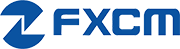Watchlist
JPY Pairs
Price Source# How Far is the Price From the Moving Averages?

## Price Deviation from MAs

The table below shows how far above or below a currency pair is trading from a chosen moving average (MA). The further away the price is from an MA, the bigger the difference (or deviation) between the price and the MA.

The distance between price and the moving average is expressed as a percentage (%).

A positive % value (in green) means price is trading ABOVE the moving average. The larger the % value, the further above the price is from the moving average. The more MAs that have positive (green) values, the stronger the uptrend. More green = more bullish.

A negative % value (in red) means price is trading UNDER the moving average. The larger the % value, the further below the price is from the moving average. The more MAs that have negative (red) values, the stronger the downtrend. More red = more bearish.

You can choose multiple MAs in the Filter above to quickly see just how many moving averages a currency pair is trading above (or below) and by how much.

Show Values

## Is Price Above/Below Its Moving Average?

The chart below allows you to compare multiple currency pairs and quickly see which ones are trading above or below a moving average.

Each colored "dot" represents the relationship between price and a specific moving average. The further right (left) the "dot" is from the middle 0% line, the further above (below) the price is from the moving average.

The currency pair with the furthest "dot" compared to other currency pairs listed means its price is the furthest away from that specific moving average and is showing the most trend strength.

When applying multiple "dots" to a currency pair, the more "dots" that are further away from the middle 0% line, the stronger the trend.